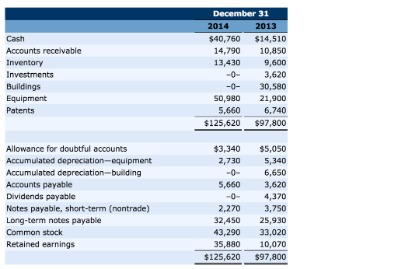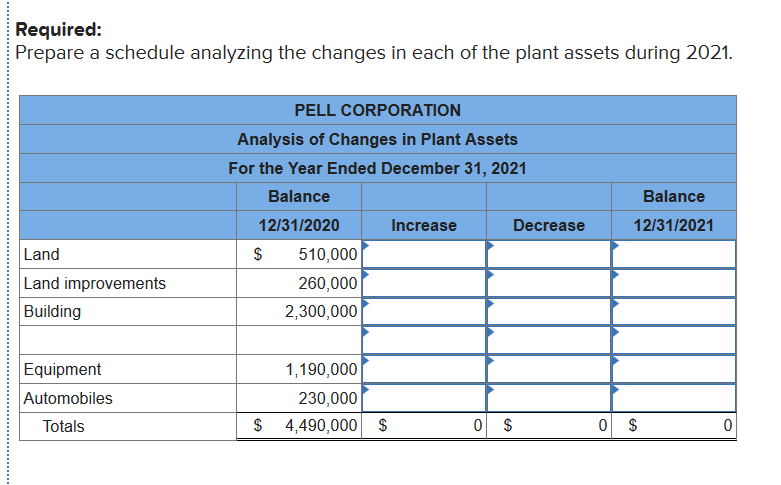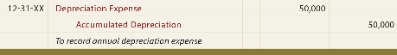# What Is The Accumulated Depreciation Formula?

Hence, the credit balance in the account Accumulated Depreciation cannot exceed the debit balance in the related asset account. After the 5-year period, if the company were to sell the asset, the account would need to be zeroed out because the asset is not relevant to the company anymore. Therefore, there would be a credit to the asset account, a debit to the accumulated depreciation account, and a gain or loss depending on the fair value of the asset and the amount received. The units-of-production depreciation method assigns an equal amount of expense to each unit produced or service rendered by the asset. Obsolescence should be considered when determining an asset’s useful life and will affect the calculation of depreciation. For example, a machine capable of producing units for 20 years may be obsolete in six years; therefore, the asset’s useful life is six years. Companies can choose a method that allocates asset cost to accounting periods according to benefits received from the use of the asset.

The cost for each year you own the asset becomes a business expense for that year. This expense is tax-deductible, so it reduces your business taxable income for the year. The years of use in the accumulated depreciation formula represent the total expected lifespan of an asset. The IRS provides data tables that can show you what the expected lifetime value of a particular asset is. Once you know the expected years of use, divide the difference between the salvage value and cost by the years of use.

This type of depreciation is a non-cash charge against the asset that is expensed on the income statement. Accumulated depreciation of an asset is an important financial metric for the business as it reduces a firm’s value on the balance sheet. The balance sheet is one of the three fundamental financial statements. The financial statements are key to both financial modeling and accounting.

## What Are The Different Ways To Calculate Depreciation?

These four methods of depreciation (straight line, units of production, sum-of-years-digits, and double-declining balance) impact revenues and assets in different ways. Accumulated depreciation represents the continuous depreciation expense of an asset over its lifetime.

The units-of-production method is calculated based on the units produced in the accounting period. Depreciation expense will be lower or higher and have a greater or lesser effect on revenues and assets based on the units produced in the period. The declining balance method is essential for recognizing most of an asset’s depreciation early in its usable life. This means that a company charges most of an asset’s depreciation expenses during the beginning years of the asset’s use, starting when the company gains the asset. In later years as the asset loses usability and value, the company assumes a reduction in depreciation of the asset’s value.

### What depreciation means?

The term depreciation refers to an accounting method used to allocate the cost of a tangible or physical asset over its useful life or life expectancy. Depreciation represents how much of an asset’s value has been used.

Typical depreciation methods can include straight line, double-declining balance, and units of production. For the double-declining balance method, revenues and assets will be reduced more in the early years of an asset’s life, due to the higher depreciation expense, and less in the later years.

## Find The Remaining Book Value Of The Asset

The asset’s cost is the original value of the asset when you first gain it, while the expected salvage value represents the total expected value of the asset after it’s no longer usable. The expected years of use represent the number of years you expect the asset to last.

Such expense is recognized by businesses for financial reporting and tax purposes. A lot of people confusedepreciationexpense with actually expensing an asset. Fixed assets are capitalized when they are purchased and reported on the balance sheet. Instead, the asset’s costs are recognized ratably over the course of its useful life with depreciation. This cost allocation method agrees with thematching principlesince costs are recognized in the time period that the help produce revenues.

• You can also accelerate depreciation legally, getting more of a tax benefit in the first year you own the property and put it into service .
• Accumulated depreciation is the total value of the asset that is expensed.
• With the units-of-production depreciation method, the amount of depreciation recorded each period depends on how much the business used the asset.
• The value of the asset on your business balance sheet at any one time is called its book value – the original cost minus accumulated depreciation.

The sum of the digits can be determined by using the formula (n2+n)/2, where n is equal to the useful life of the asset. Depreciation is defined as the expensing of an asset involved in producing revenues throughout its useful life. Depreciation for accounting purposes refers the allocation of the cost of assets to periods in which the assets are used . Depreciation expense affects the values of businesses and entities because the accumulated depreciation disclosed for each asset will reduce its book value on the balance sheet. Generally the cost is allocated as depreciation expense among the periods in which the asset is expected to be used.

## How To Calculate Accumulated Depreciation: Double

Apply the rate to the book value of the asset and ignore salvage value. At the point where book value is equal to the salvage value, no more depreciation is taken. Since the accumulated account is a balance sheet account, it is not closed at the end of the year and the \$2,000 balance is rolled to the next year. At the end of year two, Leo would record another \$2,000 of expense bringing the accumulated total to \$4,000. This annual entry would be recorded every year until the truck is fully depreciated. In other words, the accumulated account equals the fixed asset account.Accumulated depreciation is the total amount of depreciation assigned to a fixed asset over its useful life. The accumulated depreciation for Year 1 of the asset’s ten-year life is \$9,500. Since we are using straight-line depreciation, \$9,500 will be the depreciation for each year. However, the accumulated depreciation is shown in the following table since it is the sum of the asset’s depreciation. The amount of accumulated depreciation for an asset will increase over time, as depreciation continues to be charged against the asset.

## Understanding Methods And Assumptions Of Depreciation

This presentation allows investors and creditors to easily see the relative age and value of the fixed assets on the books. It also gives them an idea of the amount of depreciation costs the company will recognize in the future. Sum-of-years-digits depreciation is determined by multiplying the asset’s depreciable cost by a series of fractions based on the sum of the asset’s useful life digits. Sum-of-years digits is a depreciation method that results in a more accelerated write off of the asset than straight line but less than double-declining balance method.

It is usually reported as a single line item, but a more detailed balance sheet might list several accumulated depreciation accounts, one for each fixed asset type. Accumulated depreciation is the total depreciation for a fixed asset that has been charged to expense since that asset was acquired and made available for use. The accumulated depreciation account is an asset account with a credit balance . To calculate depreciation expense, use double the straight-line rate. For example, suppose a business has an asset with a cost of 1,000, 100 salvage value, and 5 years useful life. Since the asset has 5 years useful life, the straight-line depreciation rate equals (100% / 5) or 20% per year.

## Divide Annual Depreciation To Get Monthly Depreciation

Depreciation is an accounting method of allocating the cost of a tangible asset over its useful life and is used to account for declines in value over time. As an example, Company ABC bought a piece of equipment for \$250,000 at the start of the year. The equipment’s residual value is \$25,000, with an expected useful life of 10 years. The yearly depreciation expense using straight-line depreciation would be \$22,500 per year.Assume that a company purchased a delivery vehicle for \$50,000 and determined that the depreciation expense should be \$9,000 for 5 years. Each year the account Accumulated Depreciation will be credited for \$9,000. Since this is a balance sheet account, its balance keeps accumulating. Therefore, after three years the balance in Accumulated Depreciation will be a credit balance of \$27,000 and the vehicle’s book value will be \$23,000 (\$50,000 minus \$27,000).

## Declining Balance Method

Accumulated depreciation can shield a portion of a business’s income from taxes. Learn accounting fundamentals and how to read financial statements with CFI’s free online accounting classes. A depreciation schedule is required in financial modeling to link the three financial statements in Excel. Salvage value is the estimated book value of an asset after depreciation. It is an important component in the calculation of a depreciation schedule. Straight line basis is the simplest method of calculating depreciation and amortization, the process of expensing an asset over a specific period. It is important to note that an asset’s book value does not indicate the vehicle’s market value since depreciation is merely an allocation technique.

Small businesses have fixed assets that can be depreciated such as equipment, tools, and vehicles. For each of these assets, accumulated depreciation is the total depreciation for that asset up to and including the current accounting period. Accumulated depreciation is the total amount of depreciation expense allocated to each capital asset since the time that asset was put into use by a business. In essence, it’s the total amount of depreciation of an asset up to the point in that asset’s life. For each accounting period, an asset’s depreciation is added to the beginning accumulated depreciation balance. It is considered a non-cash expense because the recurring monthly depreciation entry does not involve a cash transaction. Because of this, the statement of cash flows prepared under the indirect method adds the depreciation expense back to calculate cash flow from operations.

• David Kindness is a Certified Public Accountant and an expert in the fields of financial accounting, corporate and individual tax planning and preparation, and investing and retirement planning.
• Net book value is the cost of an asset subtracted by its accumulated depreciation.
• The accumulated depreciation of an asset is also necessary to determine the taxable gain on the sale of an asset.
• A lot of people confusedepreciationexpense with actually expensing an asset.

Accumulated Depreciation is also the title of the contra asset account. Accumulated Depreciation is credited when Depreciation Expense is debited each accounting period.

A company reports accumulated depreciation below an asset’s original cost on the balance sheet and reports the asset’s book value adjacent to or below accumulated depreciation. As accumulated depreciation grows each period, the asset’s book value declines. At the end of the asset’s life when it has been fully depreciated, its book value equals its salvage value. In the previous example, at the end of five years, the car’s accumulated depreciation will be \$15,000 and its book value will be \$10,000. Accumulated depreciation appears on the balance sheet as a reduction from the gross amount of fixed assets reported.

## Accumulated Depreciation

The depreciation expense in an accounting period equals the number of units the asset produces during the period times the depreciation expense per unit. Accumulated depreciation equals the depreciation expense in the current period plus all depreciation taken in previous periods. Using the previous example, assume you drove 20,000 miles the first year and 15,000 miles the second.David has helped thousands of clients improve their accounting and financial systems, create budgets, and minimize their taxes. To calculate the sum of the years, you need to know the projected useful life and then add these together.

Generated by expenses involved in the earning of the accounting period’s revenues. Depreciation expense can be calculated in a variety of ways; the method chosen should be appropriate to the asset type, the asset’s expected business use, and its estimated useful life. You must calculate depreciation on capital assets every year, so you can include this depreciation cost on your business tax return.

• It is considered a non-cash expense because the recurring monthly depreciation entry does not involve a cash transaction.
• Machinery wears out, computers become obsolete, and they are expensed as their value approaches zero.
• This helps companies decide how they want to invest their funds due to depreciation affecting assets’ value.
• David has helped thousands of clients improve their accounting and financial systems, create budgets, and minimize their taxes.
• Accumulated depreciation is a credit balance on the balance sheet otherwise known as a contra account.

In business, companies record depreciation expenses every accounting period on a monthly, quarterly or yearly basis and need it to understand how accumulated depreciation expenses affect revenues. These depreciation expenses add up, or accumulate, to become the beginning accumulated depreciation balances for each new accounting period. Depreciation expenses appear on the income statement during the recording period, while accumulated depreciation shows up on the balance sheet under related capitalised assets. You’ll note that the balance increases over time as depreciation expenses are added.

The value of the asset on your business balance sheet at any one time is called its book value – the original cost minus accumulated depreciation. Book value may be related to the price of the asset if you sell it, depending on whether the asset has residual value. After you’ve calculated the straight-line depreciation, you can calculate its rate by dividing one by the asset’s lifespan years. Using the previous example, if the computer’s lifespan is six years, the straight-line depreciation rate would be 1 / 6 or 0.16. Multiply by 100 to determine this as a percentage—16% of the original value for each year of the asset’s lifetime. Accumulated amortization and accumulated depletion work in the same way as accumulated depreciation; they are all contra-asset accounts.